# N-body hollow shell gravity simulation

I first started seriously researching cosmology because while reading popular science articles on the internet, the dark matter hypothesis kept coming up.  They insisted that the only way to explain the fact that galaxies have flat rotation curves out to their perimeters was because there was a dark matter halo of particles enveloping every galaxy that added the extra mass to maintain the constant velocity.  This idea did not sit well with me for several reasons, (largely their insistence on shutting down any criticism of the theory).  As the theory goes, visible material (stars and gas) can adequately explain the rotation curves out to a certain radius of the galaxy, but after that you need additional previous unobserved matter to add enough mass to satisfy the equation m = v^2r/g. They are treating the halo as if it were a hollow spherical body, and one of the central tenets of the theory is that a star rotating around the center of the galaxy is only effected by the portion of the halo that is inside their orbit, and they are not affected by the portion of the halo outside of their orbit.  But it seemed to me that if an object was on the inside of a hollow sphere, if it were near to the interior wall of the sphere it would get pulled towards the interior wall.   After reading up about Newton’s shell theorem, they assured me that it would not be pulled away anywhere, that indeed, all of the gravitational forces are cancelled out within the sphere, that a test particle placed at any spot within the shell would remain unmoved.

They used the cones graphic to explain, but in all the demonstrations they never took a full account of all the material that makes up the hollow shell and demonstrated that it all did in fact balance out.

But, I decided I needed to prove it to myself.  So, I built an N-body hollow shell gravity simulation that would take into account all of the gravitational attraction of the material in the sphere.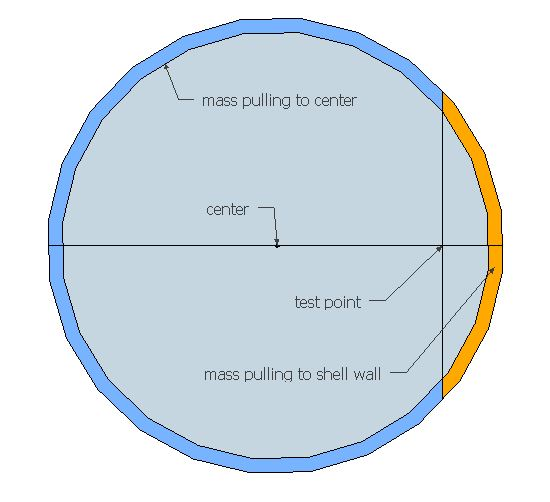The idea is pretty straightforward, the simulation is a grid, which for computational purposes treats material that would be evenly distributed matter into discrete cells, you are then able to calculate the distance and forces acting on you cell of interest and the grid that surrounds it.  Then you would shift the location of the cell of interest to gather the gravitational profile for all distances from the center of the grid all the way to the edge of it, and plot those on a line graph.

So I start by making a grid with left to right being fixed distances, and up/down being the way to change the location of the cell of interest relative to the grid. Calculate the distance from the cell of interest to all the other cells.  This is done dynamically, so when r changes, (the radius of the cell of interest) all the distances update.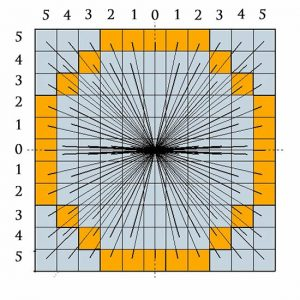I then compute the gravitational force between the green cell and all the other cells.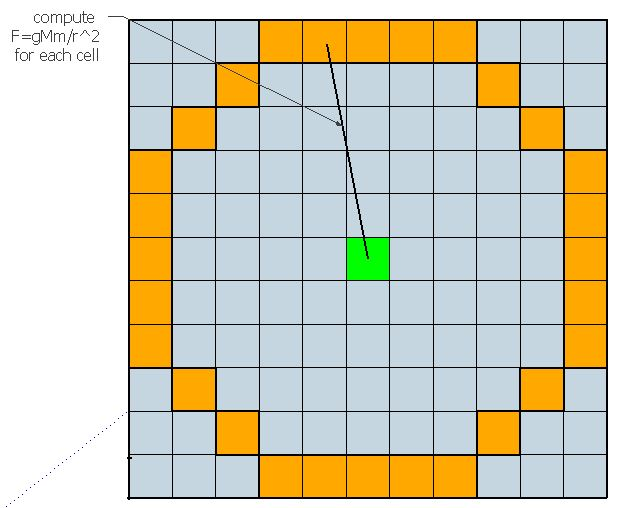Since we are trying to ascertain the sum of forces pulling out and the sum of forces pulling in, it is necessary to resolve all the vector forces in those two directions.  That is, most of the cells are not in direct line up and down with the green cell.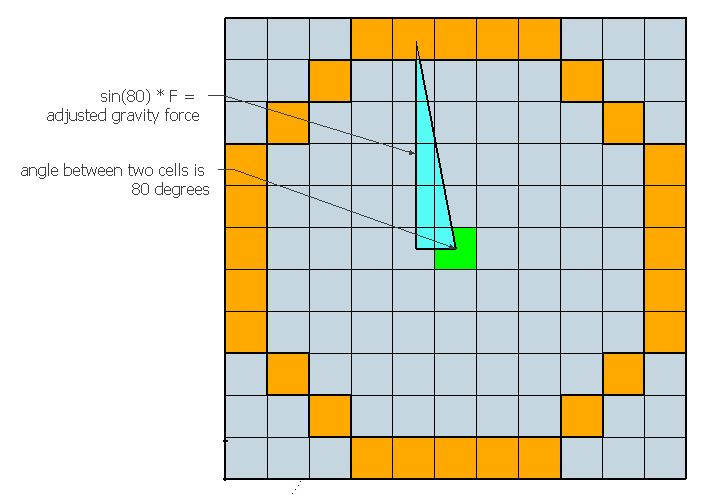I do this by taking the sine of the angle between the cell of interest and the cell I am calculating to and multiplying that by the F calculated previously.

I then need to determine which cells are actually within the ring formation, because those are the only cells with anything in them.  (This ring will later be used to build a sphere).  The ring is established at some arbitrary distance, and it is comprised of all cells with distance greater than r1, and less than r2.

To then build a sphere out of a ring, I took each cell which is in the ring and calculating its distance from the centerline, multiplied that distance by 2*pi to form a slice of a sphere.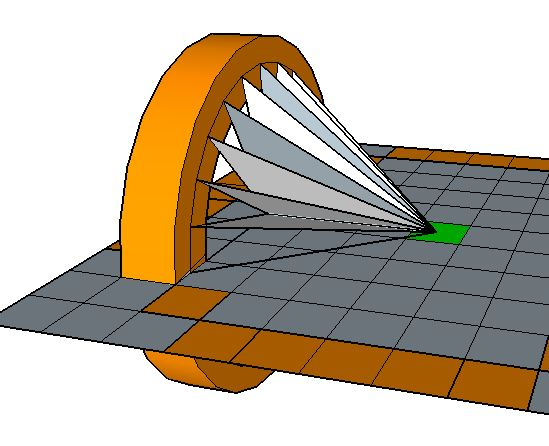Because our cell of interest is exactly centered with respect to the slice of sphere, the left/right, and up/down vector forces are balanced, and the only force left is pulling straight forward or straight behind.  That is, the resolved vector force between the cell of interest and every point in this slice of the sphere is the same, therefore we can simply take the adjusted force F previously calculated and multiply that by 2*pi*r and that yields the total gravitational force of that cross-section of hollow sphere.  Then do that for all cells within the ring.

All of the resultant forces are summed, and as we move the green cell of interest, the forces pulling it out will be compared against the forces pulling it toward the center, and we will determine if the forces really do cancel out.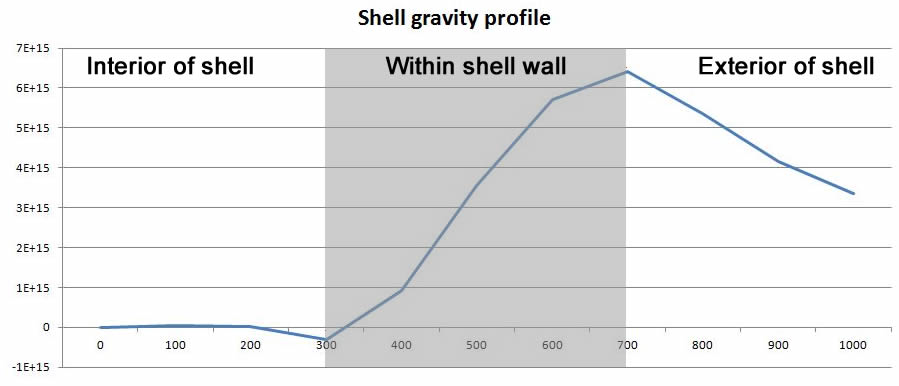The result is the line graph shown here.  In this graph the grid is 2000 light years across, with a shell composed of 1,100 stars.  The inner radius of the shell is 400 light years and the outer radius is 700 light years.  Within the interior of the shell the forces very nearly do cancel out, but as you get closer to the interior wall of the shell the pull towards the wall is greater than the pull towards the center (this is indicated by the line dipping below the 0 on the Y-axis).  And granted it is much weaker than the force outside of the shell, about 1/20th, but it is not zero. You then have a (nearly) linearly increasing force as you pass through the wall of the shell.  And outside of the shell as r increases there is the expected Keplerian decline in gravitational force F (I checked and it is the same as if the hollow shell were a single point of mass in the center).

The preceding graph is from a small scale spreadsheet that I built just for demonstration purposes for this write-up, and for someone to be able to decipher my methods, that is why this graph is so choppy and low-resolution–it is from a grid that is only 20×20, and outputs only 11 data points for the line graph–you can hardly even tell that is a Keplerian decline.  However, my main spreadsheet is 400 rows and 400 columns, so 160,000 cells, and it outputs 200 data points for the line graph.  It would be almost impossible for someone to figure out what the hell was going on in there without being walked through step-by-step, (and it is very slow for the excel macro actually to run through all the data, about 7 minutes per run on my laptop)

But here is what a similar run looks like on the bigger grid.  (The forces are flipped from the graph above)  Here the spreadsheet represents a galaxy-sized grid 150,000 light years across, with a hollow shell of approximately 6 billion solar masses, with an inner radius of 45,000 light years, and outer radius of 52,500 light years.  Here you see the same features–flat line until you reach the inner wall of the shell, then linear increase in F as you pass through the shell, then Keplerian decline in F.  However, you may note the very slight upturn in the line as it approaches the shell wall.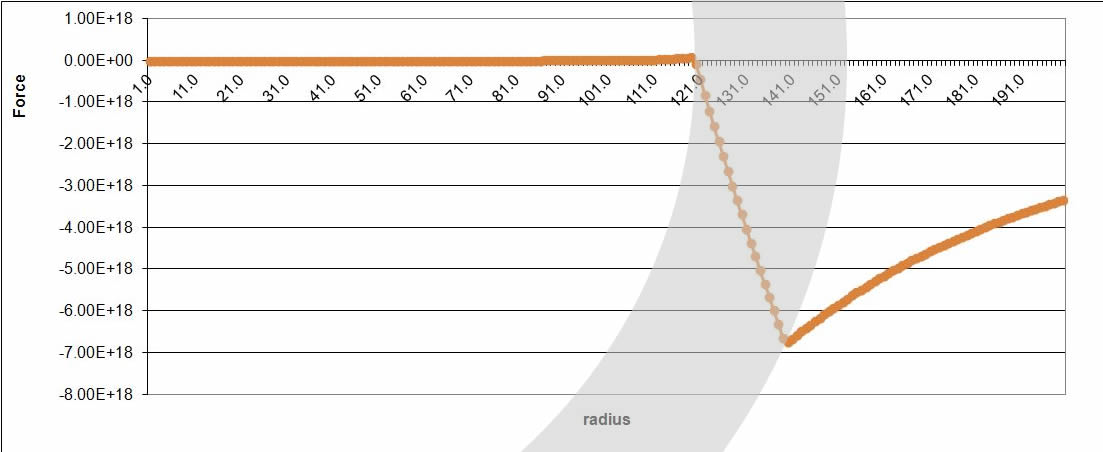If we zoom in a little closer, we can see this in greater detail, there is a very clear increase in F as it approaches the inner wall of the shell.  Compared to the gravitational forces outside the shell, those on the interior are relatively minor–the force outside is a about 90 times stronger in this case, but since they are completely separate environments, that is irrelevant.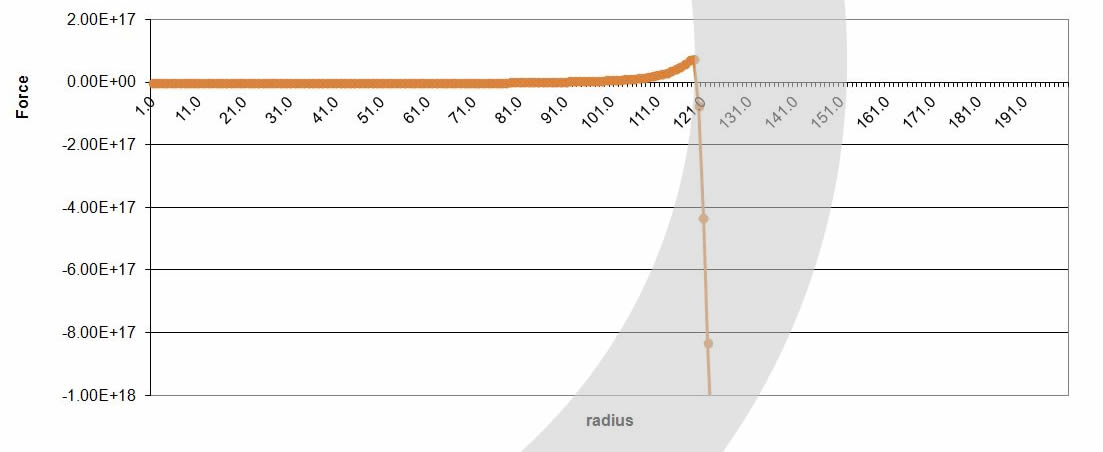Then if we zoom in still closer, we can really start to see what is going on inside the hollow shell.  There appear to be three points at which the gravitational forces completely cancel out–one at the very center of the interior of the shell, one at 0.6 of the inner radius (here it is 27,000 light years, or point 72), and one at the the very inner wall of the hollow sphere.  At all other locations, gravity is not zero.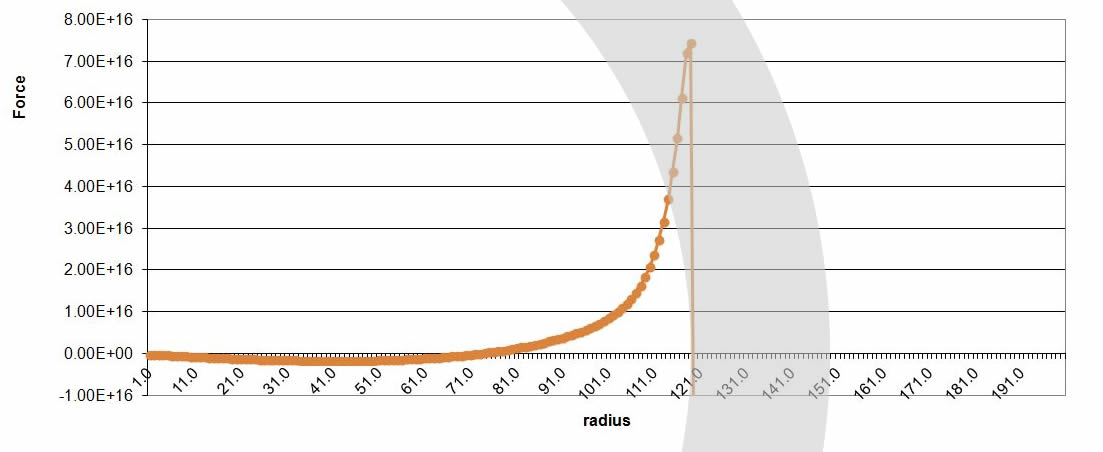Between 0 and 0.6 (0 – 72 on graph) of the inner radius there is a very, very slight pull to the center–nearly zero, it would take about 20 billion years to pull a single star to the center of the hollow sphere, but it appears it would do so in the fullness of time. (This effect is so slight that it could be due to rounding error in excel, I have no way to double check that, but at the r = 0 point it is balanced within a few thousand N, so I don’t think the rounding error can be that high)

Material placed between 0.6 and 1 (72 – 119 on graph) of the inner radius experiences much stronger gravitational forces.  The force on the inside is strong enough so that all matter within about 4,000 light years of the interior wall (110 on the graph above) will be pulled up to the shell wall within 2 billion years.  And eventually the rest of the material between 0.6 and 1 of inner radius will get pulled to the inner wall as well, though much slower.

The distance between cells in this grid is 375 light years, so the forces would likely be much stronger as you got even closer to the inner wall, but I cannot compute them with the current setup.

So, what is the final analysis?  First, I would really like for someone to go through my spreadsheet to see if I did anything wrong–I have checked everything over a hundred times, so if there is an error in calculation, I don’t know what it could be that would show all the features that would be expected of a hollow shell, but have one additional feature of non-zero-summing right at the interior wall of the shell as I predicted.  Barring an error in calculation, it appears that the forces inside of a hollow sphere do not completely cancel out, and that the shell theorem is not totally accurate.  It is not as wrong as I my intuition suggested–in fact it is nearly 100% correct, but not quite–but >99%.

But it should be noted that there is very little publicly available information about constructing N-body gravity simulations, so I am not even sure if I did any of it right, I was just sort of making it up as I went along.

It should also be noted that this hollow shell formation of stars is a completely artificial construct that could not occur in nature, and if created would immediately begin to collapse inward.  If this hollow sphere formation of stars was rotating, the outer radius of the shell would have to be spinning at 200,000m/s to balance the inward pull, but then the material closer in would be flung out (because it only needs to be going 20,000m/s to balance the inward pull), and the top and bottom would still collapse towards the center, and it would flatten to a rotating disc.  There appears to be now way to  balance such a structure.  What is supposed to prevent a dark matter halo from collapsing in the same way?

Stay tuned for more!